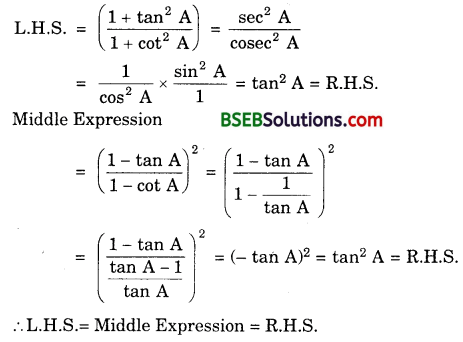# Bihar Board Class 10th Maths Solutions Chapter 8 Introduction to Trigonometry Ex 8.4

Bihar Board Class 10th Maths Solutions Chapter 8 Introduction to Trigonometry Ex 8.4 Textbook Questions and Answers.

## BSEB Bihar Board Class 10th Maths Solutions Chapter 8 Introduction to Trigonometry Ex 8.4Question 1.
Express the trigonometric ratios sin A, sec A and tan A in terms of cot A.
Solution:
Consider a ∆ ABC, in which ∠B = 90°.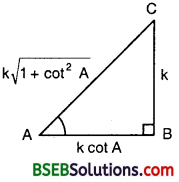Base = AB
Perp. = BC
and Hyp. = AC
∴ cot A = $$\frac{\text { Base }}{\text { Perp }}$$ = $$\frac { AB }{ BC }$$
or $$\frac { AB }{ BC }$$ = cot A = $$\frac { cot A }{ 1 }$$
Let AB = k cot A and BC = k.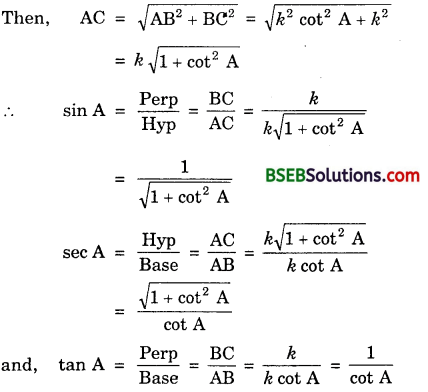Question 2.
Write the other trigonometric ratios of A in terms of sec A.
Solution:
Consider a ∆ ABC, in which ∠B = 90°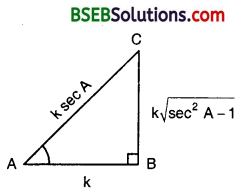For ∠A, we have :
Base = AB,
Perp = BC
Hyp = AC.
∴ sec A = $$\frac{\text { Hyp }}{\text { Base }}$$ = $$\frac { AC }{ AB }$$
or $$\frac { AC }{ AB }$$ = sec A = $$\frac { sec A }{ 1 }$$
Let AB = k and AC = k sec A.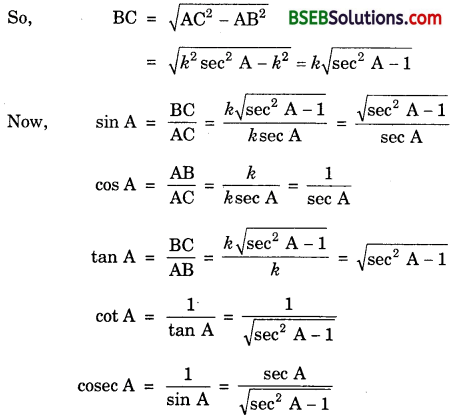Question 3.
Evaluate :
(i) $$\frac{\sin ^{2} 63^{\circ}+\sin ^{2} 27^{\circ}}{\cos ^{2} 17^{\circ}+\cos ^{2} 73^{\circ}}$$
(ii) sin 25° cos 65° + cos 25° sin 65
Solution:
(i) Here, sin 63° = sin (90° – 27°) = cos 27°
and cos 17° = cos (90° – 73°) = sin 73°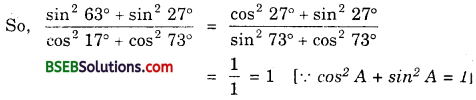(ii) sin 25° cos 65° + cos 25° sin 65°
= sin (90° – 65°) cos 65° + cos (90° – 65°) sin 65°
= cos 65° cos 65° + sin 65° sin 65°
= cos² 65° + sin² 65° = 1

Question 4.
Choose the correct option. Justify your choice :
(i) 9 sec² A – 9 tan² A =
(A) 1
(B) 9
(C) 8
(D) 0
(ii) (1 + tan θ + sec θ) (1 + cot θ – cosec θ) =
(A) 0
(B) 1
(C) 2
(D) none of these
(iii) (sec A + tan A)(1 – sin A) =
(A) sec A
(B) sin A
(C) cosec A
(D) cos A
(iv) $$\frac{1+\tan ^{2} A}{1+\cot ^{2} A}$$
(A) sec² A
(B) – 1
(C) cot² A
(D) none of these
Solution:
(i) (B), because
9 sec² A – 9 tan² A = 9 (sec² A – tan² A) = 9 x 1 = 9

(ii) (C), because
(1 + tan θ + sec θ )(1 + cot θ – cosec θ)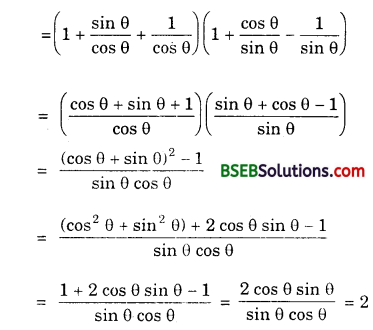(iii) (D), because
(sec A + tan A)(1 – sin A) =(iv) (D), becauseQuestion 5.
Prove the following identities, where the angles involved are acute angles for which the expressions are defined :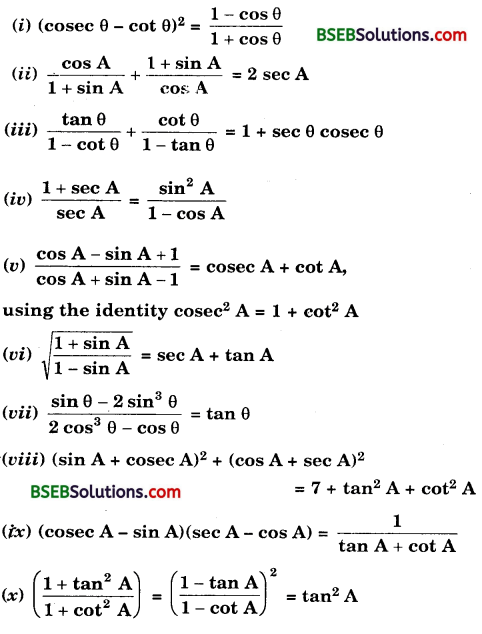Solution:
(i) We have :
L.H.S. = (cosec θ – cot θ)²

(ii) We have :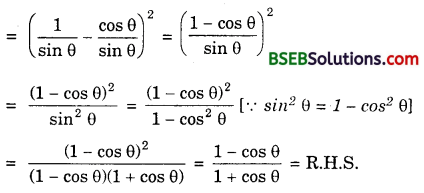(ii) We have :(iii) We have :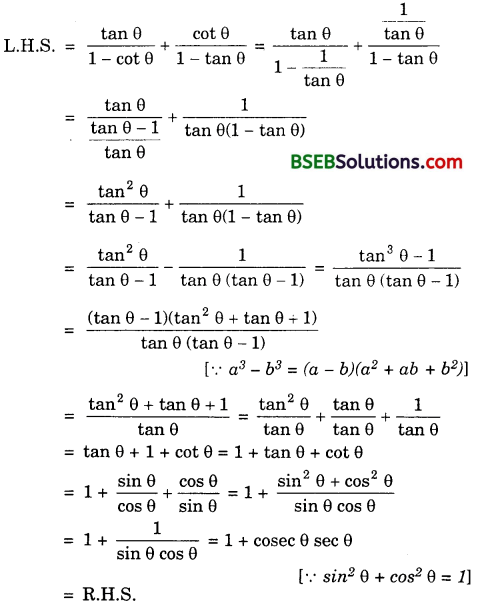(iv) We have :(v) We have :(vi) We have :(vii) We have :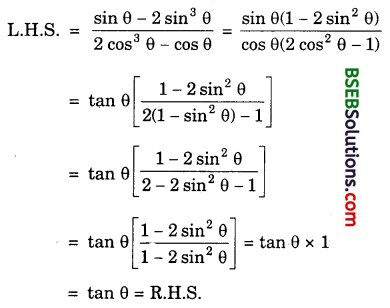(viii) We have :
L.H.S. = (sin A + cosec A)² + (cos A + sec A)²
= (sin² A + cosec² A + 2 sin A cosec A) + (cos² A + sec2 A + 2 cos A sec A)
= (sin² A + cosec² A + 2 sin A.$$\frac{1}{\sin A}$$) + (cos² A + sec2 A + 2 cos A. $$\frac{1}{\cos A}$$)
= (sin²A + cosec² A + 2) + (cos² A + sec² A + 2)
= sin²A + cos² A + cosec² A + sec² A + 4
= 1 + (1 + cot² θ) + (1 + tan² A) + 7 + tan² A + cot² A [ ∵ cosec² A = 1 + cot² A and sec² A = 1 + tan² A]
= R.H.S.

(ix) We have :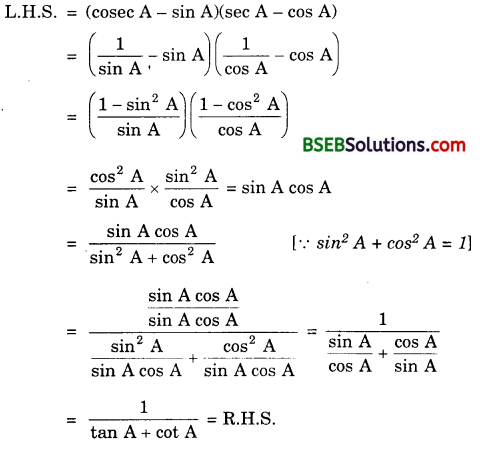(x) We have :